# ALGEBRA FX 2.0 PLUS

Close

• The colors may differ slightly from the original.

Past Model

## Features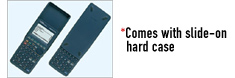• Large display (128 × 64 dots)
• Algebra Applications (Computer Algebra System, Algebra, Tutor)
• Graphic functions and Graph solve functions
• Dynamic graph
• Dual graph (Graph and Table, Graph and Graph)
• Conic section graph
• Complex functions
• List function and list-based statistics
• Statistic calculations and graphs
• Graph solve
• Integrations
• Differential and quadratic differential calculations
• BASIC-like program functions
• Linear equations from 2 to 30 unknowns
• 2 to 30 order equations
• Matrix operations with complex numbers
• Base-calculations/conversions
• Add-in application with Flash Memory
• Includes a connecting cable for data transfer between two units
• Data communication (requires optional FA-124USB for connecting with PC)

## Main Functions

### Algebra Applications

#### Computer Algebra System (CAS)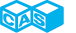Using the Computer Algebra System (CAS), students can factor expressions, find limits of functions and calculate derivatives, integration and Taylor series expressions.
The CAS is a CASIO original algebra system that was devised through the cooperation of CASIO engineers, Profession John Kenelly, and other math instructors. It directly incorporates advice and suggestions from math teachers.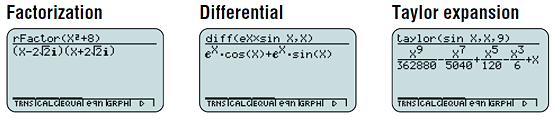#### Algebra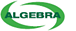Easy equation manipulation by students
The Algebra application makes it possible for students to expand and simplify equations on their own as they derive solutions. After learning to solve problems using the Tutor application (see below), students can use the Algebra application to master the steps of solving algebra problems

Easy-to-follow steps guide students to the solution." src="/media/cal/images/scientific/graphic_with_cas/afx20p/img04.gif">

#### Tutor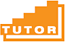Like having your own personal tutor always on hand to guide you along the way!

The TUTOR application guides student to the final solution, much like a teacher does in the classroom. The TUTOR application has three modes.

• Auto
• Manual
• Verify

## Specifications

•Dot matrix display High-resolution screen provides beautiful looking graphs every time.

•Icon menu Specify the operation you want to perform by selecting an icon or inputting a number.

•List based STAT-data editor Viewing and editing of input data in list format, showing data groups (x-data, y-data, frequency) and surrounding data.

•Data communication with a personal computer Allows data communication with a personal computer.